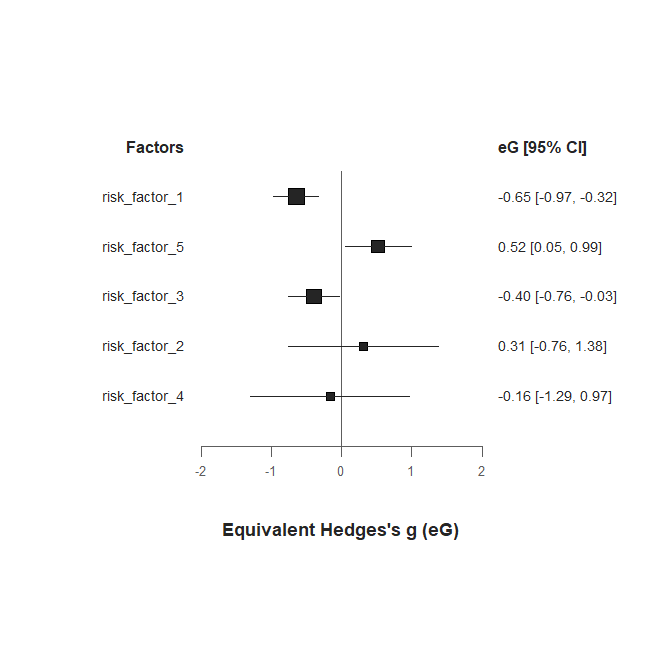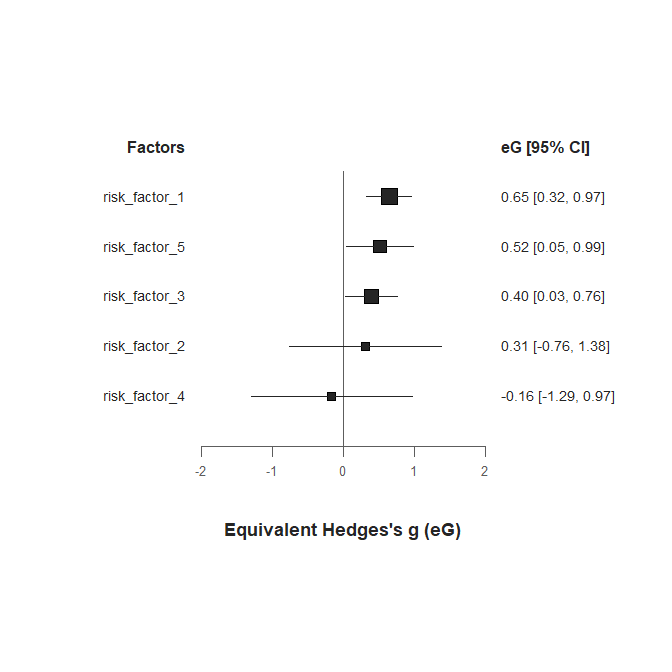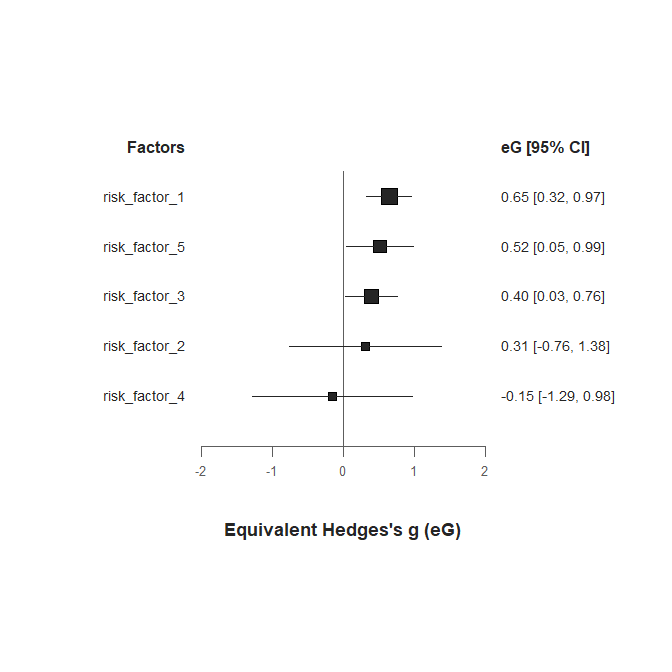# Introduction

The purpose of this vignette is to show how to format your dataset so that it can be passed to the different functions of the metaumbrella package. One of the specificities of the functions of this package lies in the fact that they do not include any argument to identify the name of the different columns of your dataset. This choice was made to facilitate the use of the functions by limiting the number of arguments. Consequently, a number of formatting rules - such as the name of the columns or the modalities of certain variables - cannot be changed.

In this document, we present a step-by-step description of how you should proceed to obtain a well formatted dataset.

# Raw data

df.train

# Use of the ‘view.errors.umbrella’ function

The first column of the dataset contains some indications that mimic those that can be done during data extraction. To format any dataset, you must follow the guidelines in the manual of the package and you can verify that your dataset is correctly formatted using the view.errors.umbrella function. This function has been created to help you formatting your dataset. Let’s apply this function on this training dataset.

errors <- view.errors.umbrella(df.train)
## ERROR:
## - The following required variables are missing:  meta_review, factor, author, year, measure

The function identifies that several columns that cannot be left empty are not included in the dataset. The information needed for the factor, author, year and measure columns are stored in the dataset but under other column names than those expected. The meta_review column is not included in the dataset. This column should contain identifiers for the different meta-analyses included in the review (e.g., the name of the first author of each meta-analysis).

# rename columns
names(df.train)[names(df.train) == "risk_factor"] <- "factor"
names(df.train)[names(df.train) == "author_study"] <- "author"
names(df.train)[names(df.train) == "year_publication_study"] <- "year"
names(df.train)[names(df.train) == "type_of_effect_size"] <- "measure"

df.train$meta_review[df.train$factor %in% c("risk_factor_1", "risk_factor_2", "risk_factor_3")] <- "Smith (2020)"
df.train$meta_review[df.train$factor %in% c("risk_factor_4")] <- "Jones (2018)"
df.train$meta_review[df.train$factor %in% c("risk_factor_5")] <- "De Martino (2015)"

After having renamed the columns and created the meta_review column, we rerun the view.errors.umbrella function.

errors <- view.errors.umbrella(df.train)
## ERROR:
## - Measure cannot be empty or NA.

The function returns a new error message and returns a dataframe containing only problematic rows. The error message indicates that some rows have a missing measure, and the dataframe helps to identify the problematic rows in your dataset. When looking more closely at the data, we can see that all the prooblematic rows have means, SD and sample size for the two groups. This information allows to calculate a SMD. We will thus request to use this effect size for these rows.

df.train[is.na(df.train$measure), ]$measure <- "SMD"

Then, we re-apply the view.errors.umbrella function to see if new error messages occurred.

errors <- view.errors.umbrella(df.train)
## ERROR:
## - For SMD, SMC, MD and G the number of cases and controls is mandatory
## - For OR measure, one group between (n_cases, n_controls) / (n_exp, n_nexp) / (n_cases_exp, n_cases_nexp, n_controls_exp, n_controls_nexp) has to be indicated.
## - For HR measure, the number of cases is mandatory
## - For RR measure, one group between (n_cases, n_controls) / (n_cases_exp, n_cases_nexp, n_controls_exp, n_controls_nexp) has to be indicated.
## - For IRR measure, only one group between (n_cases), and (n_cases_exp, n_cases_nexp) can be empty, not both.
## - Some repeated studies (author and year) in the same factor do not have any 'multiple_es' value.
## - SMD measure is not associated with sufficient information to run the umbrella review.
## - HR measure is not associated with sufficient information to run the umbrella review.
## - OR measure is not associated with sufficient information to run the umbrella review.
## - RR measure is not associated with sufficient information to run the umbrella review.
## - IRR measure is not associated with sufficient information to run the umbrella review.
## WARNING:
## - No warning

New error messages are now displayed! Sometimes, when you resolve some error messages, new ones appear. This is because the view.errors.umbrella works step-by-step to avoid producing an overwhelming number of error messages at the same time. The new error messages concern the sample sizes. When looking at the data, we can see that information on sample sizes is present but not stored in columns with the names expected by the functions of the metaumbrella We thus have to rename all of them.

names(df.train)[names(df.train) == "number_of_cases_exposed"] <- "n_cases_exp"
names(df.train)[names(df.train) == "number_of_cases_non_exposed"] <- "n_cases_nexp"
names(df.train)[names(df.train) == "number_of_controls_exposed"] <- "n_controls_exp"
names(df.train)[names(df.train) == "number_of_controls_non_exposed"] <- "n_controls_nexp"

names(df.train)[names(df.train) == "number_of_participants_exposed"] <- "n_exp"
names(df.train)[names(df.train) == "number_of_participants_non_exposed"] <- "n_nexp"

names(df.train)[names(df.train) == "number_of_cases"] <- "n_cases"
names(df.train)[names(df.train) == "number_of_controls"] <- "n_controls" 
errors <- view.errors.umbrella(df.train)
## ERROR:
## - Some repeated studies (author and year) in the same factor do not have any 'multiple_es' value.
## - SMD measure is not associated with sufficient information to run the umbrella review.
## - HR measure is not associated with sufficient information to run the umbrella review.
## - IRR measure is not associated with sufficient information to run the umbrella review.
## WARNING:
## - No warning

It is indicated that the value and 95% CI of the HR and the time of the IRR are missing. Again, even if the information is present in the dataset, the function is missing it because the column names are not appropriate.

names(df.train)[names(df.train) == "effect_size_value"] <- "value"
names(df.train)[names(df.train) == "low_bound_ci"] <- "ci_lo"
names(df.train)[names(df.train) == "up_bound_ci"] <- "ci_up"
names(df.train)[names(df.train) == "time_disease_free"] <- "time" 
errors <- view.errors.umbrella(df.train)
## ERROR:
## - Some repeated studies (author and year) in the same factor do not have any 'multiple_es' value.
## - SMD measure is not associated with sufficient information to run the umbrella review.
## WARNING:
## - No warning

Only two error messages are now displayed. One regards the information about the calculation of the SMD. When looking at the corresponding rows, we can see that it is stated in the column_errors that the means and SD are missing. Column names of means / sd have to be changed to be identified by the function.

names(df.train)[names(df.train) == "mean_of_intervention_group"] <- "mean_cases"
names(df.train)[names(df.train) == "mean_of_control_group"] <- "mean_controls"
names(df.train)[names(df.train) == "sd_of_intervention_group"] <- "sd_cases"
names(df.train)[names(df.train) == "sd_of_control_group"] <- "sd_controls" 

# Multilevel data

errors <- view.errors.umbrella(df.train)
## ERROR:
## - Some repeated studies (author and year) in the same factor do not have any 'multiple_es' value.
## WARNING:
## - No warning

Only one message error is now displayed, indicating the two studies have the same author and year of publication within the same factor. The functions of the metaumbrella package always identify studies with same author and year of publication in the same factor as a study with dependent effect sizes. When looking at the comments of the two rows highlighted, we see that a study has two effect sizes because authors have reported the effect on two distinct outcomes. This information has be indicated in the multiple_es column. Because the same sample has completed two outcomes, we have indicate this to the function using the “outcomes” value. You can also indicate the correlation between the outcomes of this study in the r column. We will fix it at .60.

df.train$multiple_es <- df.train$r <- NA

df.train[which(duplicated(paste(df.train$author, df.train$year)) | duplicated(paste(df.train$author, df.train$year), fromLast = TRUE)), ]$multiple_es <- "outcomes" df.train[which(duplicated(paste(df.train$author, df.train$year)) | duplicated(paste(df.train$author, df.train$year), fromLast = TRUE)), ]$r <- .60
errors <- view.errors.umbrella(df.train)
## Your dataset is well formatted.

The function now indicates that the dataset is ready to be passed to the functions of the package!

Let’s try some.

umb <- umbrella(df.train, mult.level = TRUE, method.var = "REML")
## Analyzing factor: risk_factor_1
## Analyzing factor: risk_factor_2
## Analyzing factor: risk_factor_3
## In factor 'risk_factor_3':
## - study: 'Thornock (2004)' contains multiple outcomes
## Analyzing factor: risk_factor_4
## Analyzing factor: risk_factor_5

A warning message indicates that the umbrella function has detected the multiple outcomes of the Thornock (2004) study.

forest(umb)Interestingly, when looking at the forest plot, we can see that the different risk factors could have an effect in opposite directions.
Let’s go back to the comments made in the original dataset to ensure we have not missed anything.

# Reverse effect size direction

df.train

We can see that it has been indicated that the risk factors 1 and 3 have effect sizes in opposite directions. To facilitate presentation of the results, we can use the reverse_es column in the dataset. This column allows to flip the direction of some effect sizes automatically. To do so, you have to indicate the value reverse in rows for which you want to flip the effect size.

df.train$reverse_es <- NA df.train[df.train$factor %in% c("risk_factor_1", "risk_factor_3"), ]$reverse_es <- "reverse" Now, we can rerun calculations and visualize the results. umb <- umbrella(df.train, mult.level = TRUE) forest(umb)As you can see, the pooled effect sizes of these two factor still have exactly the same magnitude as previously but their direction is reversed. Now, the pooled effect sizes of the 5 factors have the same meaning. # Shared control/non-exposed groups There is only one comment left to address in the original data set. It indicates that two separate articles compared the same non-exposed group to two distinct exposed groups. The participants in this group are given too much weight since they are analyzed as two separate groups. To correct this, you need to indicate that these two studies share the same non-exposed group. Doing so, the number of participants in this group will be divided by two and the two studies will be considered as independent. The effect size value and its standard error will be recalculated using the corrected sample size. df.train$shared_nexp <- NA
df.train\$shared_nexp[22:23] <- "el-Neman"

You are now ready for data analysis!

umb <- umbrella(df.train, mult.level = TRUE)
forest(umb)